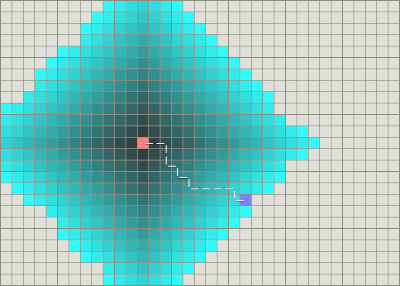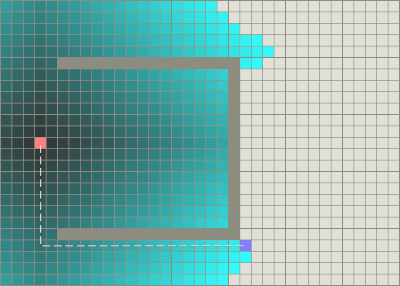### A* 演算法簡介 (A* Algorithm Brief)

A* (A-Star) 演算法是在Game中通常用來解決最短路徑(Shortest Path)問題的一種演算法. 相對於另一個知名的 Dijkstra 演算法來說, Dijkstra演算法雖然可以保證找到一條最短的路徑, 但不如A* 演算法這樣簡捷快速. 同時, Dijkstra的搜尋深度在某些情形下也容易顯得不適用. A* 演算法便是為了這些情形而出現的, 可以算是 Dijkstra演算法的一種改良.

 Dijkstra 演算法 A* 演算法 無障礙有障礙`f(n) = g(n) + h(n)`
g(n): 從啟始點到目前節點的距離
h(n): 預測目前節點到結束點的距離(此為 A* 演算法的主要評價公式)
f(n): 目前結點的評價分數

1. h(n)=0: A* 演算法這時等同 Dijkstra演算法, 並且保證能找到最短路徑
2. h(n)<目前節點到結束點的距離: A* 演算法保證找到最短路徑, h(n)越小, 搜尋深度越深
3. h(n)=目前節點到結束點的距離: A* 演算法僅會尋找最佳路徑, 並且能快速找到結果
4. h(n)>目前節點到結束點的距離: 不保證能找到最短路徑, 但計算比較快
5. h(n)與g(n)高度相關: A* 演算法此時成為 BFS (Best-First Search)

A* 演算法的虛擬碼如下:

while OPEN not empty

get node n from OPEN that has the lowest f(n)

if n is GOAL then return path

move n to CLOSED

for each n' = CanMove(n, direction)

g(n') = g(n) + 1

calculate h(n')

if n' in OPEN list and new n' is not better, continue

if n' in CLOSED list and new n' is not better, continue

remove any n' from OPEN and CLOSED

end for

end while

if we get to here, then there is No Solution

Amit's Thoughts on Path-Finding and A-Star
Creating an A* algorithm Robot for QUT SoKoBan

Shortest Path Problem
A* Search Algorithm
Dijkstra Algorithm

Core Techniques and Algorithms in Game Programming (英文)

#### 意見

1. h(n)=0: A* 演算法這時等同 Dijkstra演算法, 並且保證能找到最短路徑
2. h(n)<目前節點到結束點的距離: A* 演算法保證找到最短路徑, h(n)越小, 搜尋深度越深
3. h(n)=目前節點到結束點的距離: A* 演算法僅會尋找最佳路徑, 並且能快速找到結果
4. h(n)>目前節點到結束點的距離: 不保證能找到最短路徑, 但計算比較快
5. h(n)與g(n)高度相關: A* 演算法此時成為 BFS (Best-First Search)